# Central Limit Theorem explained in Python (with examples)By Ashutosh Dave

The Central Limit Theorem (CLT) is often referred to as one of the most important theorems, not only in statistics but also in the sciences as a whole. In this blog, we will try to understand the essence of the Central Limit Theorem with simulations in Python.

Contents

• Samples and the Sampling Distribution
• What is the Central Limit Theorem?
• Central Limit Theorem: Statement & Assumptions
• Demonstration of CLT in action using simulations in Python with examples
• The importance of the Central Limit Theorem

## Samples and the Sampling Distribution

Before we get to the theorem itself, it is first essential to understand the building blocks and the context. The main goal of inferential statistics is to draw inferences about a given Population, using only its subset, which is called the sample.

We do so because generally, the parameters which define the distribution of the population, such as the population mean $$(\mu)$$ and the population variance $$(\sigma^{2})$$, are not known.

In such situations, a sample is typically collected in a random fashion, and the information gathered from it is then used to derive estimates for the entire population.

The above-mentioned approach is both time-efficient and cost-effective for the organization/firm/researcher conducting the analysis. It is important that the sample is a good representation of the population, in order to generalize the inferences drawn from the sample to the population in any meaningful way.

The challenge though is that being a subset, the sample estimates are well, just estimates, and hence prone to error! That is, they may not reflect the population accurately.

For example, if we are trying to estimate the population mean $$(\mu)$$ using a sample mean $$(\bar x)$$, then depending on which observations land in the sample, we might get different estimates of the population with varying levels of errors.

## What is the Central Limit Theorem?

The core point here is that the sample mean itself is a random variable, which is dependent on the sample observations.

Like any other random variable in statistics, the sample mean $$(\bar x)$$ also has a probability distribution, which shows the probability densities for different values of the sample mean.

This Distribution is often referred to as the 'sampling distribution'. The following diagram summarizes this point visually: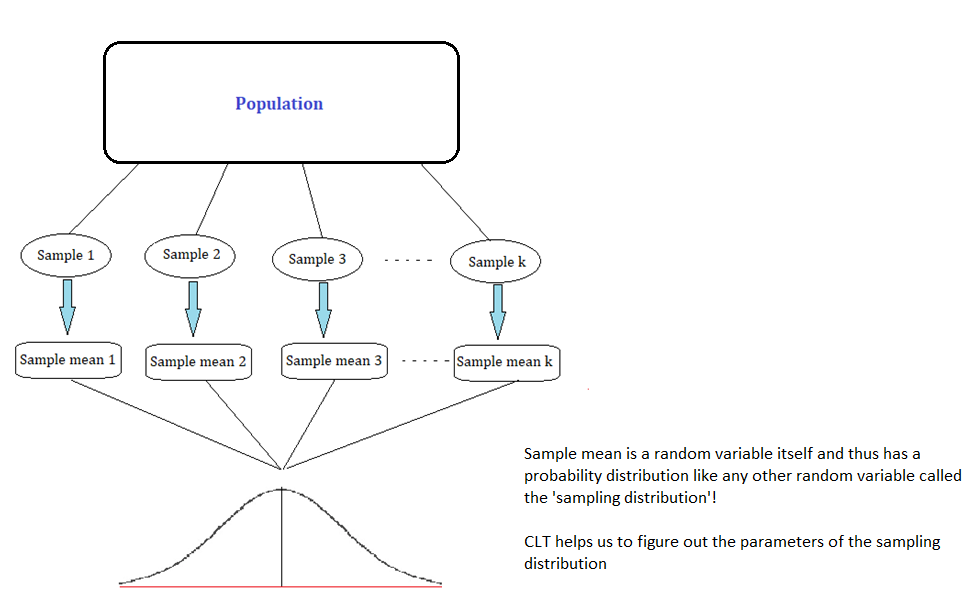The Central Limit Theorem essentially is a statement about the nature of the sampling distribution of the sample mean under some specific condition, which we will discuss in the next section.

## Central Limit Theorem: Statement & Assumptions

Suppose we are taking repeated samples of size 'n' from a population with any kind of probability distribution. Then, the Central Limit Theorem states that given a high enough sample size, the following properties hold true:

• Sampling distribution's mean = Population mean $$(\mu)$$, and
• Sampling distribution's standard deviation (standard error) = $$\sigma/√n$$, such that for n ≥ 30, the sampling distribution tends to a normal distribution for all practical purposes.

In the next section, we will try to understand the workings of the CLT with the help of simulations in Python.

## Demonstration of CLT in action using simulations in Python with examples

The main point demonstrated in this section will be that for a population following any distribution, the sampling distribution (sample mean's distribution) will tend to be normally distributed for large enough sample size.

We will consider two examples and check whether the CLT holds.

### Example 1: Exponentially distributed population

Suppose we are dealing with a population which is exponentially distributed. Exponential distribution is a continuous distribution that is often used to model the expected time one needs to wait before the occurrence of an event.

The main parameter of exponential distribution is the 'rate' parameter $$\lambda$$, such that both the mean and the standard deviation of the distribution are given by $$(1/\lambda)$$.

The following represents our exponentially distributed population: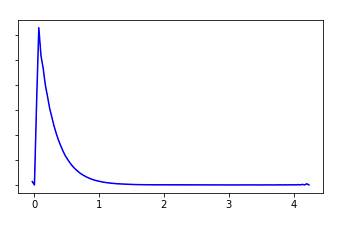f(x) = $$\cases{\lambda e^{-\lambda x} & if x> 0\cr0 & \text{otherwise}}$$

E(X) = $$1/\lambda$$ = $$\mu$$V(X) = $$1/\lambda^2$$ = $$\sigma^2$$, which means SD(X) = $$1/\lambda$$ = $$\sigma$$

We can see that the distribution of our population is far from normal! In the following code, assuming that $$\lambda$$=0.25, we calculate the mean and the standard deviation of the population:

Population mean: 4.0
Population standard deviation: 4.0

Now we want to see how the sampling distribution looks for this population. We will consider two cases, i.e. with a small sample size (n= 2), and a large sample size (n=500).

First, we will draw 50 random samples from our population of size 2 each. The code to do the same in Python is given below:

sample 1 sample 2 sample 3 sample 4 sample 5 sample 6 sample 7 sample 8 sample 9 sample 10 ... sample 41 sample 42 sample 43 sample 44 sample 45 sample 46 sample 47 sample 48 sample 49 sample 50
x1 3.308423 7.105807 0.787859 2.811602 0.255161 5.085278 7.253975 2.549191 1.318133 0.659430 ... 13.017465 10.280906 1.863208 4.000935 1.119582 1.640825 7.242127 0.807044 11.797688 4.585229
x2 2.969489 1.082994 3.382971 3.474494 8.949835 0.993594 7.335135 5.529222 3.760836 1.690919 ... 8.690013 1.468530 0.376954 0.167118 4.100110 0.255927 1.754906 3.647159 1.883523 1.101046

2 rows × 50 columns

For each of the 50 samples, we can calculate the sample mean and plot its distribution as follows: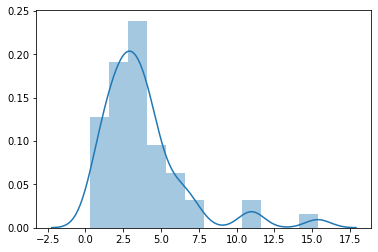We can observe that even for a small sample size such as 2, the distribution of sample means looks very different from that of the exponential population, and looks more like a poor approximation of a normal distribution, with some positive skew.

In case 2, we will repeat the above process, but with a much larger sample size (n=500):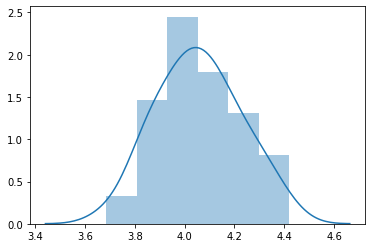The sampling distribution looks much more like a normal distribution now as we have sampled with a much larger sample size (n=500).

Let us now check the mean and the standard deviation of the 50 sample means:

Sample means
sample 1 4.061534
sample 2 4.052322
sample 3 3.823000
sample 4 4.261559
sample 5 3.838798

We can observe that the mean of all the sample means is quite close to the population mean $$(\mu = 4)$$.

Similarly, we can observe that the standard deviation of the 50 sample means is quite close to the value stated by the CLT, i.e., $$( \sigma / \sqrt{n})$$ = 0.178.

0.18886796530269118
0.17888543819998318

Thus, we observe that the mean of all sample means is very close in value to the population mean itself.

Also, we can observe that as we increased the sample size from 2 to 500, the distribution of sample means increasingly starts resembling a normal distribution, with mean given by the population mean $$\mu$$ and the standard deviation given by $$(\sigma / \sqrt{n})$$ , as stated by the Central Limit Theorem.

### Example 2: Binomially distributed population

In the previous example, we knew that the population is exponentially distributed with parameter $$\lambda$$=0.25.

Now you might wonder what would happen to the sampling distribution if we had a population which followed some other distribution say, Binomial distribution for example.

Would the sampling distribution still resemble the normal distribution for large sample sizes as stated by the CLT?

Let's test it out. The following represents our Binomially distributed population (recall that Binomial is a discrete distribution and hence we produce the probability mass function below):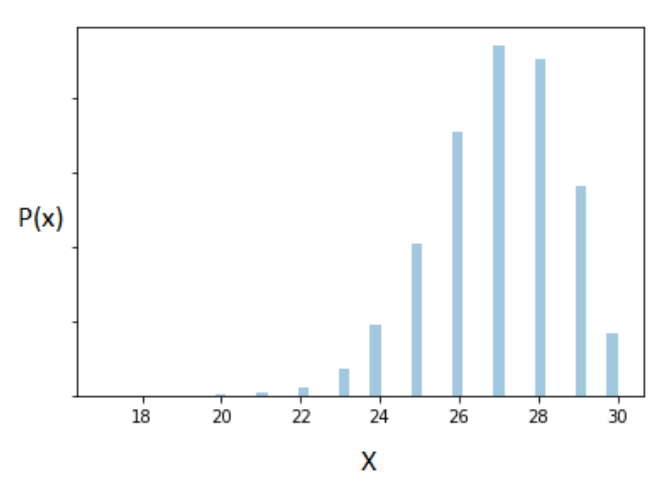P(x) = $$\cases{\binom k x (p)^x(1-p)^{1-x} & if x = 0, 1, 2,..., k \cr0 & \text{otherwise}}$$
where, $$0\leq p\leq 1$$

E(X) = kp = $$\mu$$

V(X) = kp(1-p), which means SD(X) = $$\sqrt{kp(1-p)}$$ = $$\sigma$$

As before, we follow a similar approach and plot the sampling distribution obtained with a large sample size(n = 500) for a Binomially distributed variable with parameters k=30 and p = 0,9 :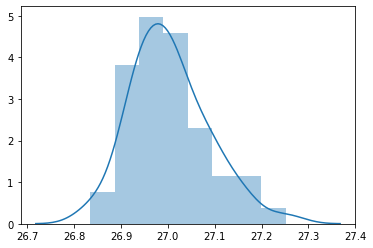For this example, as we assumed that our population follows a Binomial distribution with parameters k = 30 and p =0.9.

Which means if CLT were to hold, the sampling distribution should be approximately normal with mean = population mean = $$\mu= 27$$ and standard deviation = $$\sigma/\sqrt{n}$$ = 0.734.
26.99175999999999
0.06752975459086173

And the CLT holds again, as can be seen in the above plot.

The sampling distribution for a Binomially distributed population also tends to a normal distribution with mean $$\mu=$$ and standard deviation $$\sigma/\sqrt{n}$$ for large sample size.

## The importance of the Central Limit Theorem

The larger point demonstrated in the above two examples is that irrespective of the shape of the original population distribution, the sampling distribution will tend to a normal distribution as stated by the CLT.

In the previous two examples, we knew all the parameters of our populations. However, even if we did not know the population parameters, we can reverse engineer the estimates for the population parameters assuming that the sampling distribution is normally distributed. Therein lie the importance and appeal of the Central Limit Theorem.

Many important techniques which are used frequently in statistics and research such as hypothesis testing and confidence intervals emanate and rely on the Central Limit Theorem.

Thus, it would not be wrong to say that the CLT forms the backbone of inferential statistics in many ways, and has rightly earned its place as one of the most consequential theorems in all of the sciences!

## Conclusion

Central Limit Theorem is a really powerful statement as it implies that even if we do not know how the population is distributed, we can still approximate the distribution of the sample mean to be normal (given large enough sample size), and which in turn can help us to estimate parameters for the population itself!

In this blog, we have learnt the essence of the Central Limit Theorem. In the next blog, we will see how the Central Limit Theorem is used in the world of Finance. Till then, keep learning!

Disclaimer: All investments and trading in the stock market involve risk. Any decisions to place trades in the financial markets, including trading in stock or options or other financial instruments is a personal decision that should only be made after thorough research, including a personal risk and financial assessment and the engagement of professional assistance to the extent you believe necessary. The trading strategies or related information mentioned in this article is for informational purposes only.

Visit blog to download

This post first appeared on Best Algo Trading Platforms Used In Indian Market, please read the originial post: here

# Share the post

Central Limit Theorem explained in Python (with examples)

×

# Subscribe to Best Algo Trading Platforms Used In Indian Market

Get updates delivered right to your inbox!

×